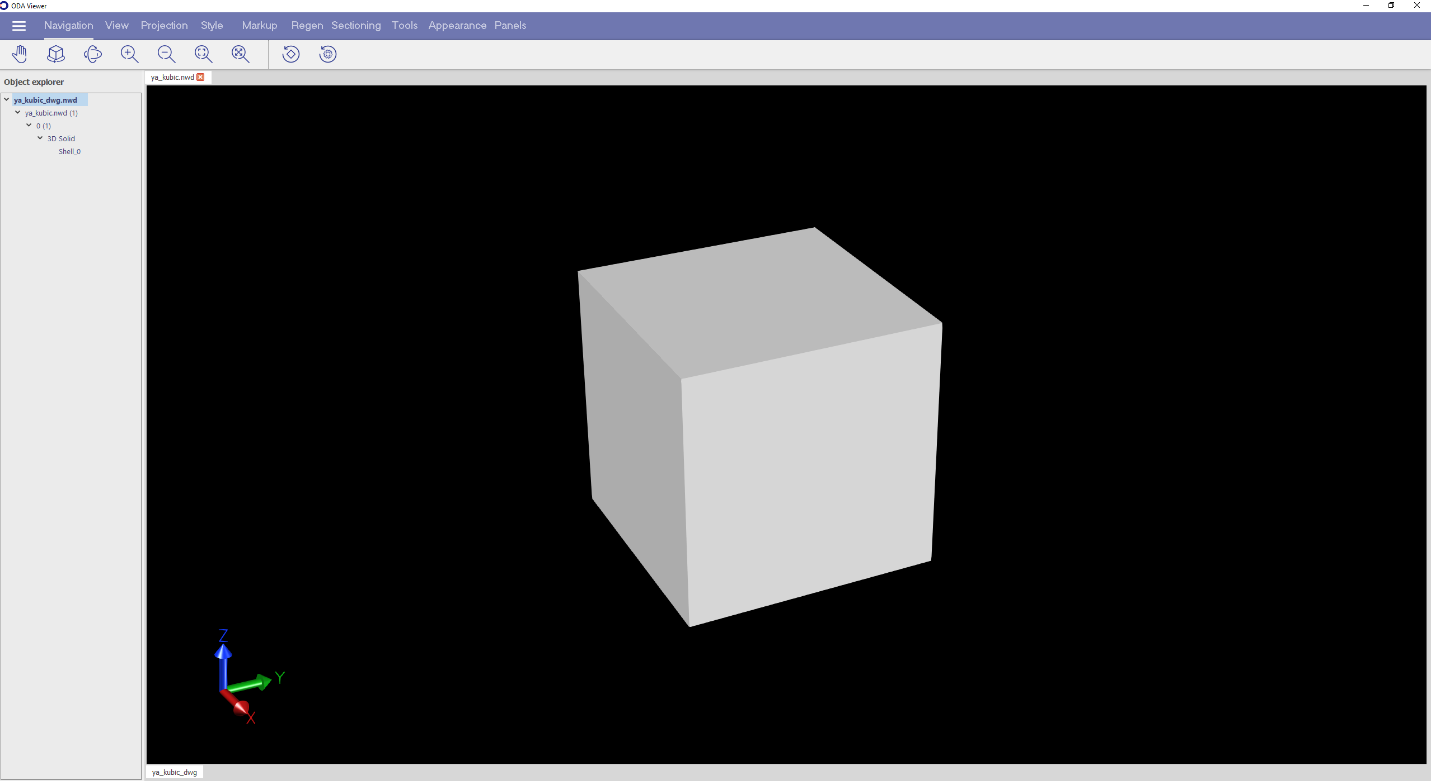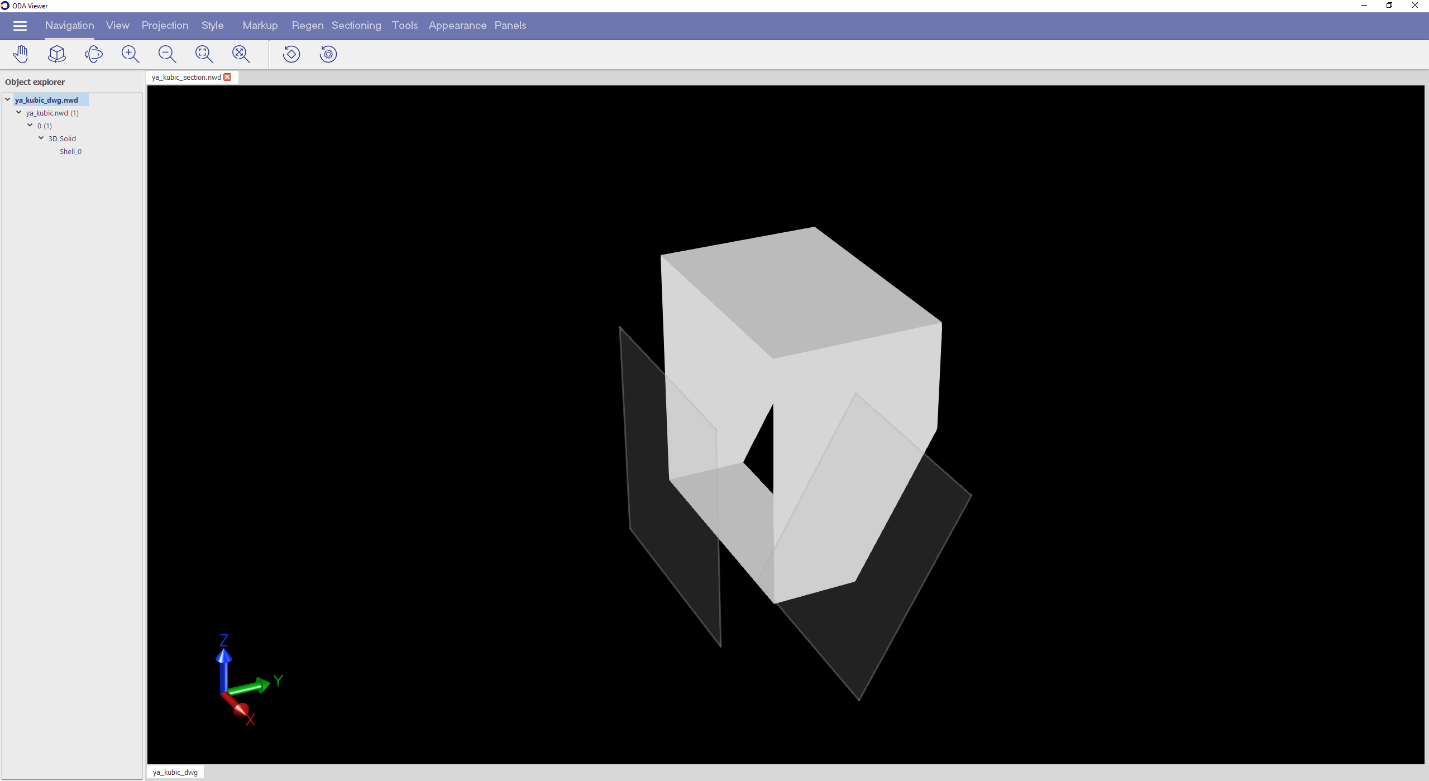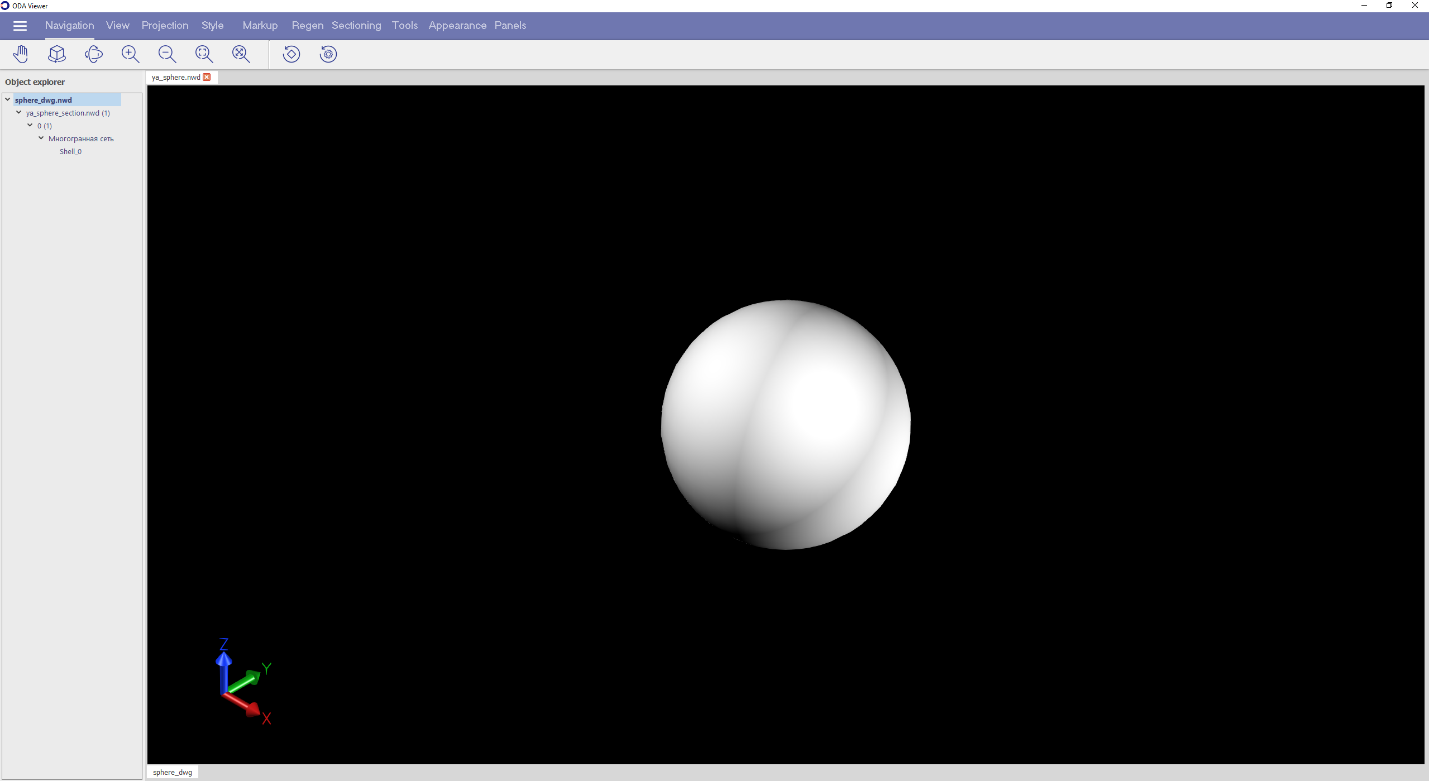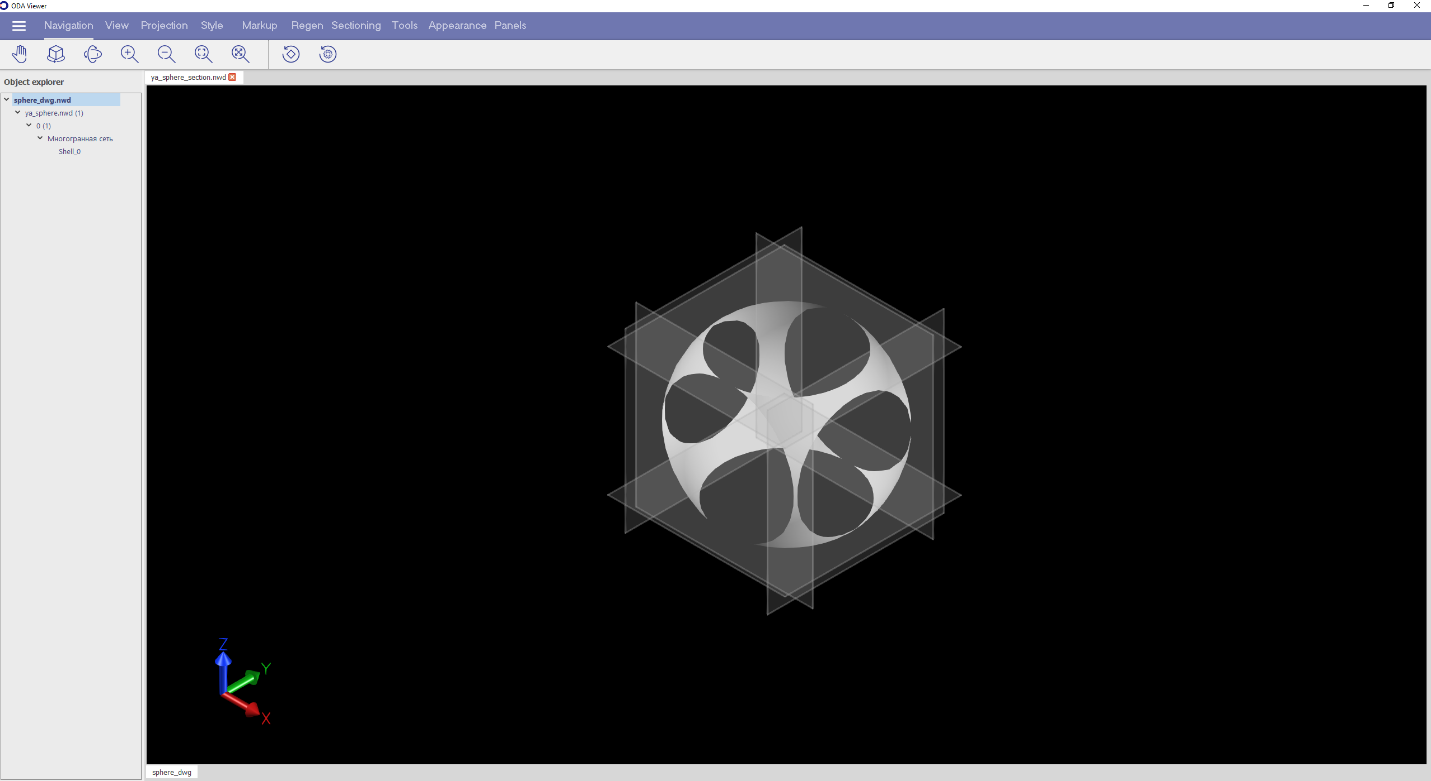# BimNv SDK: Working with Sections

February 12, 2021

This article describes how to work with sections of a view that are divided by clipping planes.

BimNv SDK provides access to section objects for the current view and for saved views. You can get them using these methods:

``````OdNwClipPlaneSetPtr OdNwDatabase::getCurrrentViewClippingPlanes() const;
OdNwClipPlaneSetPtr OdNwSavedViewpoint::getClippingPlanes() const;``````

First, enable section mode for the selected view. This can be checked using the following method:

``bool OdNwClipPlaneSet::isEnabled() const;``

An object of the OdNwClipPlaneSet class has a set of six clipping planes and can be in either plane mode or box mode.

You can get a clipping plane by its index from a set using the following method:

``OdResult OdNwClipPlaneSet::getClipPlaneByIndex(OdNwClipPlane& clipPlane, OdUInt32 idx);``

From each object of the OdNwClipPlane class, you can get the following information about it:

• Type of plane alignment:
• Alignment to view
• Alignment to back
• Alignment to front
• Alignment to left
• Alignment to right
• Alignment to top
• Alignment to bottom
• Alignment to surface
• Alignment to line
• Custom alignment
• State of the plane:
• Enabled for clipping
• Has default clipping behavior (depends on the section mode of plane or box)
• Object of the OdGePlane class with geometry data (position, normal)

You can get an object of the OdGePlane class from a clipping plane using the following method:

``OdGePlane OdNwClipPlane::getPlane() const``

#### Plane Mode

In plane mode, the planes that participate in a section have the enabled state, and you can get all geometry data for the plane (position, normal or axes) from the corresponding OdGePlane object. The planes with the default state do not participate in the section.

The following sample code illustrates working with sections in plane mode.

``````OdNwClipPlane plane;
//get the plane with index 3
if (eOk == pClipPlaneSet->getClipPlaneByIndex(plane, 3))
{
//get the plane state (DEFAULT - switched off or ENABLED - switched on)
NwClipPlaneState::Enum state = plane.getState();
if (state == NwClipPlaneState::ENABLED)
{
//get the section plane parameters
OdGePlane gePlane = plane.getPlane();
OdGePoint3d origin;
OdGeVector3d axis1, axis2;
gePlane.getCoordSystem(origin, axis1, axis2);
}
}``````

The following two images illustrate the model without sections and the section of the same model with two clipping planes in plane (enabled) mode.#### Box Mode

In box mode, all planes have the default state, so the geometric data that can be retrieved with the OdGePlane object is not relevant. For each of all six planes, it is necessary to calculate the values of the position and normal using a transformation matrix, which you can get using the following method:

``OdGeMatrix3d OdNwClipPlaneSet::getTransform() const``

The following sample code illustrates working with sections in box mode.

``````if (pClipPlaneSet->getMode() == NwClipPlaneSetMode::CL_BOX)
{
//get the transformation matrix of sections
OdGeMatrix3d matrix = pClipPlaneSet->getTransform();
auto xAxis = matrix.getCsXAxis();
auto yAxis = matrix.getCsYAxis();
auto zAxis = matrix.getCsZAxis();

OdGePlane planeTop = OdGePlane(OdGePoint3d(0, 0, 0.5).transformBy(matrix), yAxis, xAxis);
OdGePlane planeBottom = OdGePlane(OdGePoint3d(0, 0, -0.5).transformBy(matrix), xAxis, yAxis);
OdGePlane planeFront = OdGePlane(OdGePoint3d(0, -0.5, 0).transformBy(matrix), zAxis, xAxis);
OdGePlane planeBack = OdGePlane(OdGePoint3d(0, 0.5, 0).transformBy(matrix), xAxis, zAxis);
OdGePlane planeLeft = OdGePlane(OdGePoint3d(-0.5, 0, 0).transformBy(matrix), yAxis, zAxis);
OdGePlane planeRight = OdGePlane(OdGePoint3d(0.5, 0, 0).transformBy(matrix), zAxis, yAxis);

//if the coordinate system is left hand, it is required to reverse the normals of planes
if (xAxis.crossProduct(yAxis).dotProduct(zAxis) < 0)
{
planeTop.reverseNormal();
planeBottom.reverseNormal();
planeFront.reverseNormal();
planeBack.reverseNormal();
planeLeft.reverseNormal();
planeRight.reverseNormal();
}
}``````

The above code, in box mode for sections, is executed in the getPlanesForActiveMode() method:

``OdResult OdNwClipPlaneSet::getPlanesForActiveMode(OdArray<OdGePlane>& aPlanes) const``

The getPlanesForActiveMode() method helps to get a set of planes with geometric data. In plane mode, this method returns OdGePlane objects for clipping with the enabled state, and for clipping with the default state it returns the default OdGePlane object.

In the following two images, you can see the model without sections and the same model with six clipping planes in box (default) mode.